• ABV-Indian Institute of Information Technology And Management, Gwalior

# Data Structures

Abhay Chaturvedi, Utkarsh Dwivedi

* * *

# DATA STRUCTURE

Data Structures are the programmatic way of storing data so that data can be used efficiently. Almost every enterprise application uses various types of data structures in one or the other way.

## Array Representation

Arrays can be declared in various ways in different languages. For illustration, let's take C array declaration.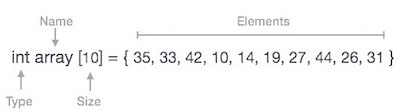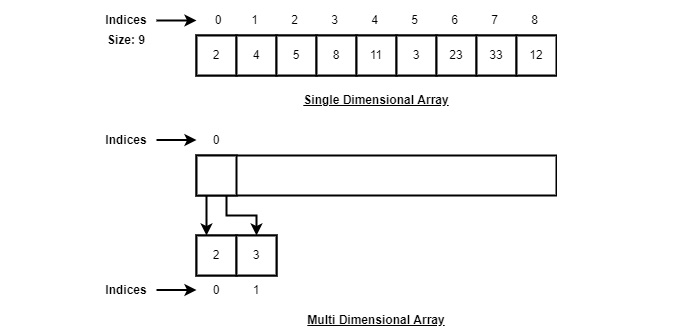As per the above illustration, following are the important points to be considered.

• Index starts with 0.
• Array length is 10 which means it can store 10 elements.
• Each element can be accessed via its index. i.e. array gives 19.

## Linked List

Visual Representation(singly, doubly linked list, stack, queue, dequeue)

Video link of linked list Implementation

Linked list can be visualized as a chain of nodes, where every node points to the next node.## Types of Linked List

Following are the various types of linked list.

• Simple Linked List − Item navigation is forward only.
• Doubly Linked List − Items can be navigated forward and backward.
• Circular Linked List − Last item contains link of the first element as next and the first element has a link to the last element as previous.

## Insertion Operation

Video link of insertion in linked list

Adding a new node in linked list is a more than one step activity. We shall learn this with diagrams here. First, create a node using the same structure and find the location where it has to be inserted.If we are inserting a node NewNode, between LeftNode and RightNode. Then point NewNode->next to RightNode.

`NewNode->next = RightNode;`

This will generate the following link between new and right node.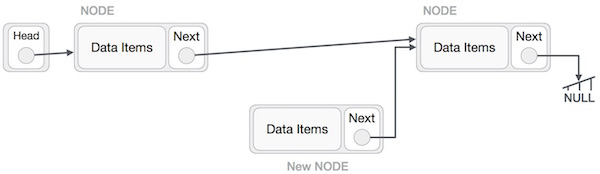Now, the next node at the left should point to the new node.

`LeftNode.next −> NewNode;`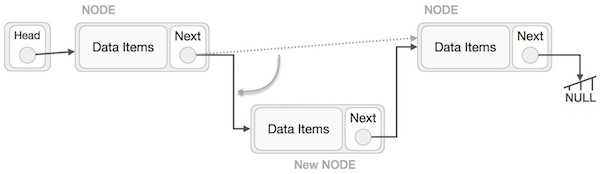This will put the new node in the middle of the two. The new list should look like thisSimilar steps should be taken if the node is being inserted at the beginning of the list. While inserting it at the end, the second last node of the list should point to the new node and the new node will point to NULL.

## Deletion Operation

Video link of deletion in linked list

Deletion is also a more than one step process. We shall learn with pictorial representation. First, locate the target node to be removed, by using searching algorithms.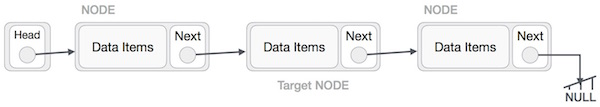The left node of the target node now should point to the right node of the target node.

`LeftNode->next = TargetNode->next;`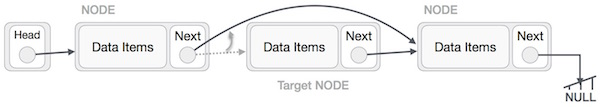We can keep that node in memory otherwise we can simply deallocate memory and wipe off the target node completely using free() function in c++.

`free(TargetNODE);`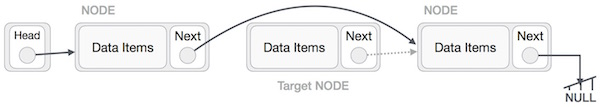## Doubly Linked List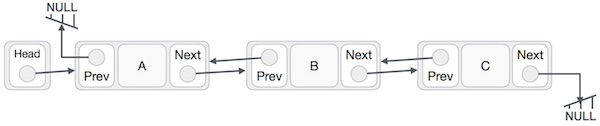As per the above illustration, following are the important points to be considered.

• Doubly Linked List contains a link element called first and last.
• Each link carries a data field(s) and two link fields called next and prev.
• Each link is linked with its next link using its next link.
• Each link is linked with its previous link using its previous link.
• The last link carries a link as null to mark the end of the list.

## Insertion Operation

Following code demonstrates the insertion operation at the beginning of a doubly linked list.

Example

```void insertFirst(int key, int data) {

//create a link

struct node *link = (struct node*) malloc(sizeof(struct node));

link->key = key;

link->data = data;

if(isEmpty()) {

//make it the last link

last = link;

} else {

//update first prev link

head->prev = link;

}

//point it to old first link

link->next = head;

//point first to new first link

head = link;

}```

## Deletion Operation

Following code demonstrates the deletion operation at the beginning of a doubly linked list.

Example

```struct node* deleteFirst() {

//save reference to first link

struct node *tempLink = head;

//if only one link

if(head->next == NULL) {

last = NULL;

} else {

head->next->prev = NULL;

}

head = head->next;

//return the deleted link

return tempLink;

}```

## Insertion at the End of an Operation

Following code demonstrates the insertion operation at the last position of a doubly linked list.

Example

```void insertLast(int key, int data) {

//create a link

struct node *link = (struct node*) malloc(sizeof(struct node));

link->key = key;

link->data = data;

if(isEmpty()) {

//make it the last link

last = link;

} else {

//make link a new last link

last->next = link;

//mark old last node as prev of new link

link->prev = last;

}

//point last to new last node

last = link;

}```

### Singly Linked List as Circular

In singly Circular linked list, the next pointer of the last node points to the first node.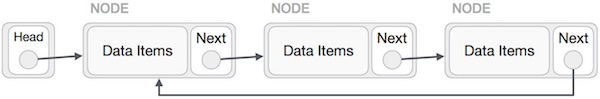## Stack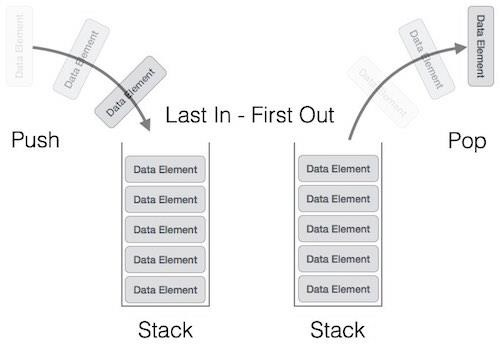A stack can be implemented by means of Array, Structure, Pointer, and Linked List. Stack can either be a fixed size one or it may have a sense of dynamic resizing. Here, we are going to implement stack using arrays, which makes it a fixed size stack implementation.

Basic Operations

• push() − Pushing (storing) an element on the stack.
• pop() − Removing (accessing) an element from the stack.
• top() − get the top data element of the stack, without removing it.
• isFull() − check if stack is full.
• isEmpty() − check if stack is empty.

### Push Operation

```void push(int data) {

if(!isFull()) {

top = top + 1;

stack[top] = data;

} else {

printf("Could not insert data, Stack is full. ");

}

}```

### Pop Operation

A Pop operation may involve the following steps −

• Step 1 − Checks if the stack is empty.
• Step 2 − If the stack is empty, produces an error and exit.
• Step 3 − If the stack is not empty, accesses the data element at which top is pointing.
• Step 4 − Decreases the value of top by 1.
• Step 5 − Returns success.
```int pop(int data) {

if(!isempty()) {

data = stack[top];

top = top - 1;

return data;

} else {

printf("Could not retrieve data, Stack is empty. ");

}

}```

## Queue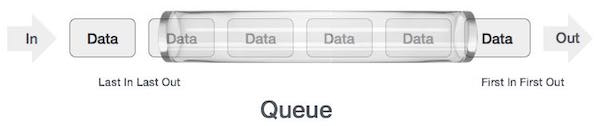As in stacks, a queue can also be implemented using Arrays, Linked-lists, Pointers and Structures. For the sake of simplicity, we shall implement queues using one-dimensional array.

Basic Operations

• enqueue() − add (store) an item to the queue.
• dequeue() − remove (access) an item from the queue.
• front() − Gets the element at the front of the queue without removing it.
• isfull() − Checks if the queue is full.
• isempty() − Checks if the queue is empty.

## Enqueue Operation

Queues maintain two data pointers, front and rear. Therefore, its operations are comparatively difficult to implement than that of stacks.

The following steps should be taken to enqueue (insert) data into a queue −

• Step 1 − Check if the queue is full.
• Step 2 − If the queue is full, produce overflow error and exit.
• Step 3 − If the queue is not full, increment rear pointer to point the next empty space.
• Step 4 − Add data element to the queue location, where the rear is pointing.
• Step 5 − return success.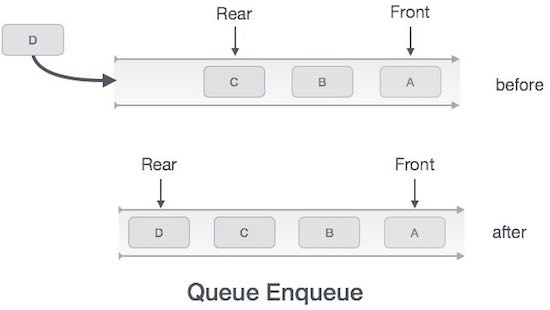Sometimes, we also check to see if a queue is initialized or not, to handle any unforeseen situations.

Example

```int enqueue(int data)

if(isfull())

return 0;

rear = rear + 1;

queue[rear] = data;

return 1;

end procedure```

## Dequeue Operation

Accessing data from the queue is a process of two tasks − access the data where front is pointing and remove the data after access. The following steps are taken to perform dequeue operation −

• Step 1 − Check if the queue is empty.
• Step 2 − If the queue is empty, produce underflow error and exit.
• Step 3 − If the queue is not empty, access the data where front is pointing.
• Step 4 − Increment front pointer to point to the next available data element.
• Step 5 − Return success.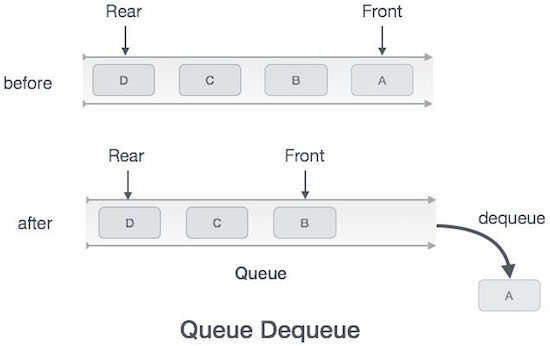Example

```int dequeue() {

if(isempty())

return 0;

int data = queue[front];

front = front + 1;

return data;

}```

# Deque

Operations on Deque:
Mainly the following four basic operations are performed on queue:

• insertFront(): Adds an item at the front of Deque.
• insertLast(): Adds an item at the rear of Deque.
• deleteFront(): Deletes an item from front of Deque.
• deleteLast(): Deletes an item from rear of Deque.
• getFront(): Gets the front item from queue.
• getRear(): Gets the last item from queue.
• isEmpty(): Checks whether Deque is empty or not.
• isFull(): Checks whether Deque is full or not.# TREE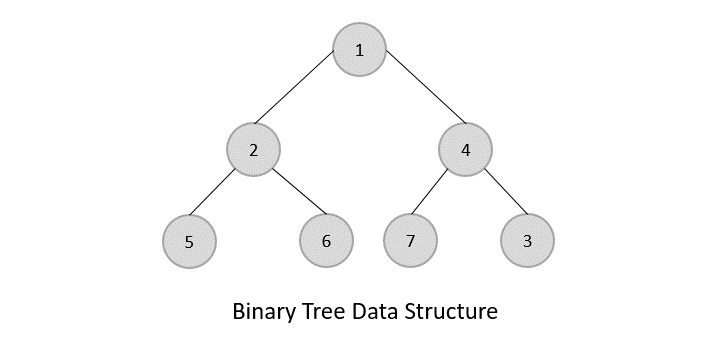Important Terms

Following are the important terms with respect to tree.

• Path − Path refers to the sequence of nodes along the edges of a tree.
• Root − The node at the top of the tree is called root. There is only one root per tree and one path from the root node to any node.
• Parent − Any node except the root node has one edge upward to a node called parent.
• Child − The node below a given node connected by its edge downward is called its child node.
• Leaf − The node which does not have any child node is called the leaf node.
• Subtree − Subtree represents the descendants of a node.
• Visiting − Visiting refers to checking the value of a node when control is on the node.
• Traversing − Traversing means passing through nodes in a specific order.
• Levels − Level of a node represents the generation of a node. If the root node is at level 0, then its next child node is at level 1, its grandchild is at level 2, and so on.
• keys − Key represents a value of a node based on which a search operation is to be carried out for a node.

## Binary Search Tree

Visual Representation(binary search tree)

Binary Search tree exhibits a special behavior. A node's left child must have a value less than its parent's value and the node's right child must have a value greater than its parent value.We're going to implement tree using node object and connecting them through references.

## Tree Node

The code to write a tree node would be similar to what is given below. It has a data part and references to its left and right child nodes.

```struct node {

int data;

struct node *leftChild;

struct node *rightChild;

};```

In a tree, all nodes share common construct.

### BST Basic Operations

The basic operations that can be performed on a binary search tree data structure, are the following −

• Insert − Inserts an element in a tree/create a tree.
• Search − Searches an element in a tree.
• Preorder Traversal − Traverses a tree in a pre-order manner.
• Inorder Traversal − Traverses a tree in an in-order manner.
• Postorder Traversal − Traverses a tree in a post-order manner.

## Traversal

Code For Traversal(inorder, preorder and post order)

Video For Traversal(inorder, preorder and post order)

There are three ways which we use to traverse a tree −

• In-order Traversal
• Pre-order Traversal
• Post-order Traversal

## In-order Traversal

In this traversal method, the left subtree is visited first, then the root and later the right sub-tree. We should always remember that every node may represent a subtree itself.

If a binary tree is traversed in-order, the output will produce sorted key values in an ascending order.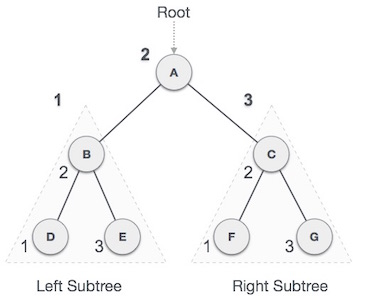We start from A, and following in-order traversal, we move to its left subtree BB is also traversed in-order. The process goes on until all the nodes are visited. The output of inorder traversal of this tree will be −

D → B → E → A → F → C → G

### Algorithm

```Until all nodes are traversed −
Step 1 − Recursively traverse left subtree.
Step 2 − Visit root node.
Step 3 − Recursively traverse right subtree.```

## Pre-order Traversal

In this traversal method, the root node is visited first, then the left subtree and finally the right subtree.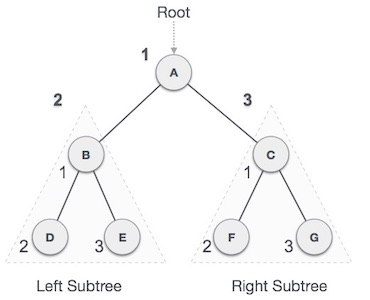We start from A, and following pre-order traversal, we first visit A itself and then move to its left subtree BB is also traversed pre-order. The process goes on until all the nodes are visited. The output of pre-order traversal of this tree will be −

A → B → D → E → C → F → G

### Algorithm

```Until all nodes are traversed −
Step 1 − Visit root node.
Step 2 − Recursively traverse left subtree.
Step 3 − Recursively traverse right subtree.
```

## Post-order Traversal

In this traversal method, the root node is visited last, hence the name. First we traverse the left subtree, then the right subtree and finally the root node.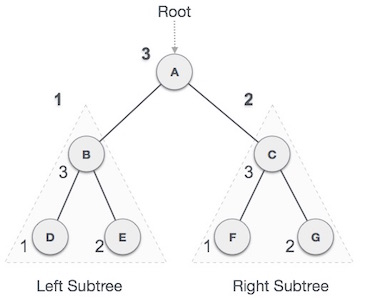We start from A, and following Post-order traversal, we first visit the left subtree BB is also traversed post-order. The process goes on until all the nodes are visited. The output of post-order traversal of this tree will be −

D → E → B → F → G → C → A

### Algorithm

```Until all nodes are traversed −
Step 1 − Recursively traverse left subtree.
Step 2 − Recursively traverse right subtree.
Step 3 − Visit root node.```

## Insertion and Search

Video For Binary Search Tree Insertion (Recursive Method)

Video For Binary Search Tree Insertion(Iterative Method)

Searching a key
To search a given key in Binary Search Tree, we first compare it with root, if the key is present at root, we return root. If key is greater than root’s key, we recur for right subtree of root node. Otherwise we recur for left subtree.

```struct node* search(struct node* root, int key)

{

// Base Cases: root is null or key is present at root

if (root == NULL || root->key == key)

return root;

// Key is greater than root's key

if (root->key < key)

return search(root->right, key);

// Key is smaller than root's key

return search(root->left, key);

}```

Insertion of a key
A new key is always inserted at leaf. We start searching a key from root till we hit a leaf node. Once a leaf node is found, the new node is added as a child of the leaf node.

```         100                               100
/           Insert 40            /
20     500    --------->          20     500
/                                /
10   30                           10   30

40```

### Implementation

```   struct node* insert(struct node* root, int data)
{
if (root == NULL)    //If the tree is empty, return a new,single node
return newNode(data);
else
{
//Otherwise, recur down the tree
if (data <= root->data)
root->left  = insert(root->left, data);
else
root->right = insert(root->right, data);
//return the (unchanged) root pointer
return root;
}
}```

# Deletion in Binary Search Tree

Video For Deletion In Binary Search Tree

When we delete a node, three possibilities arise.

1) Node to be deleted is leaf: Simply remove from the tree.

```              50                            50
/              delete(20)      /
30      70       --------->    30     70
/      /                           /
20   40  60   80                   40  60   80```

2) Node to be deleted has only one child: Copy the child to the node and delete the child

```              50                            50
/              delete(30)      /
30      70       --------->    40     70
/                            /
40  60   80                       60   80```

3) Node to be deleted has two children:

1) Find inorder successor of the node.

2) Copy contents of the inorder successor to the node and delete the inorder successor.

Note that inorder predecessor can also be used.

```              50                            60
/             delete(50)      /
40      70       --------->    40    70
/
60   80                           80```

The important thing to note is, inorder successor is needed only when right child is not empty. In this particular case, inorder successor can be obtained by finding the minimum value in right child of the node.

```
node* Delete( node* root,int value)
{
c=Search(root,value);
if(root==NULL)
return root;
else if(value< root->data)
{
root->left= Delete(root->left,value);
}
else if(value> root->data)
{
root->right= Delete(root->right,value);
}

// Node deletion
else
{
//case 1: Leaf Node
if(root->left==NULL&&root->right==NULL)    //if both childs are null
{
delete root;
root=NULL;
return root;
}
//case 2: one child
else if(root->left==NULL)                 // if left child in null
{
struct node* temp=root;
root=root->right;
delete temp;
return root;
}
else if(root->right==NULL)                // if right child is null
{
struct node* temp=root;
root=root->left;
delete temp;
return root;
}
//case 3: 2 child
else
{
struct node*temp=findMin(root->right);                    ///finding inorder succesor which is minimum of right node.
root->data=temp->data;                                    //put storing value of inorder succesor in that node
root->right=Delete(root->right,temp->data);               //now delete that temp node
}
}
return root;

}
```

## For Advanced Data Structures:-

1) https://www.codechef.com/certification/data-structures-and-algorithms/prepare#foundation